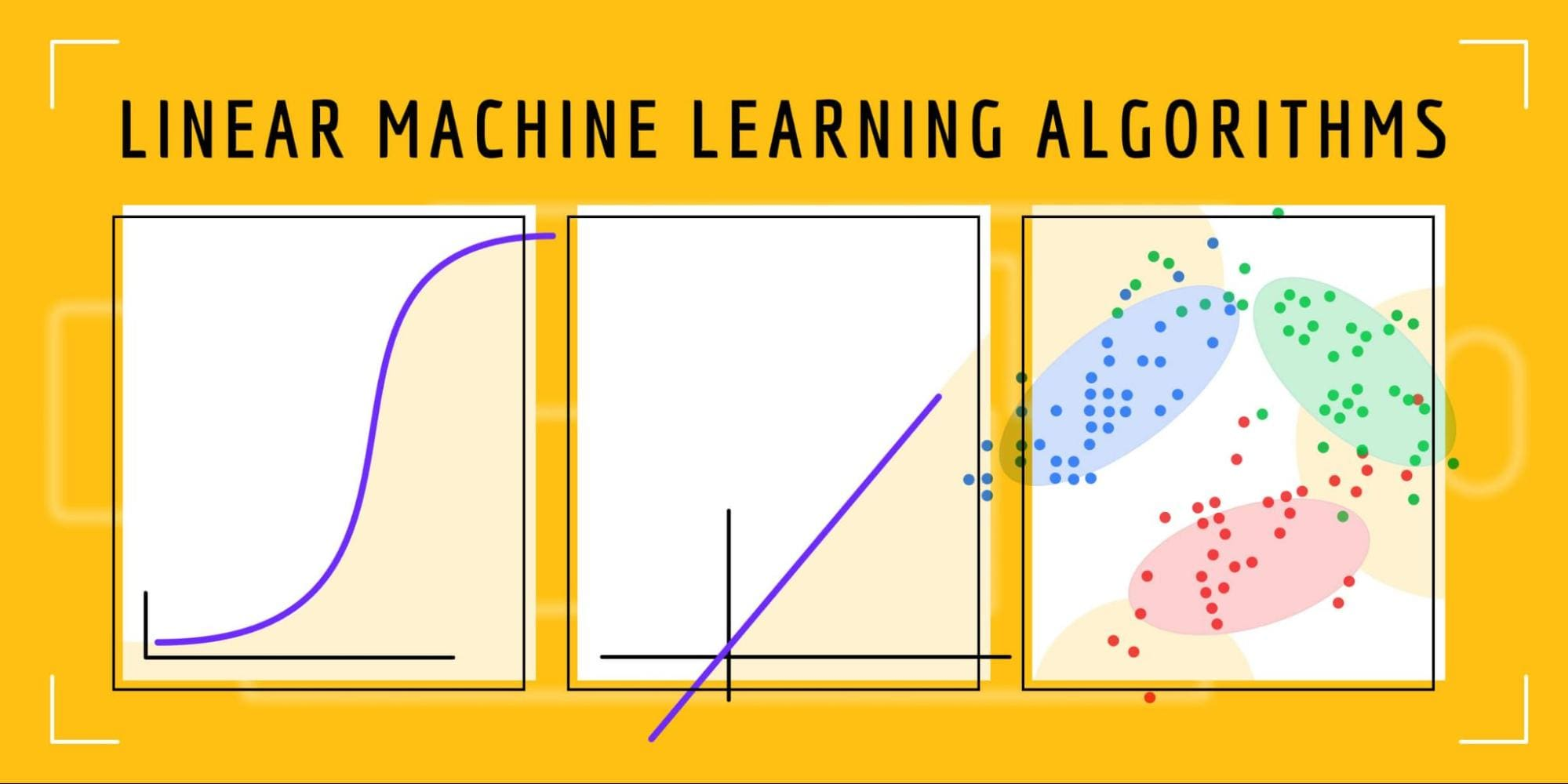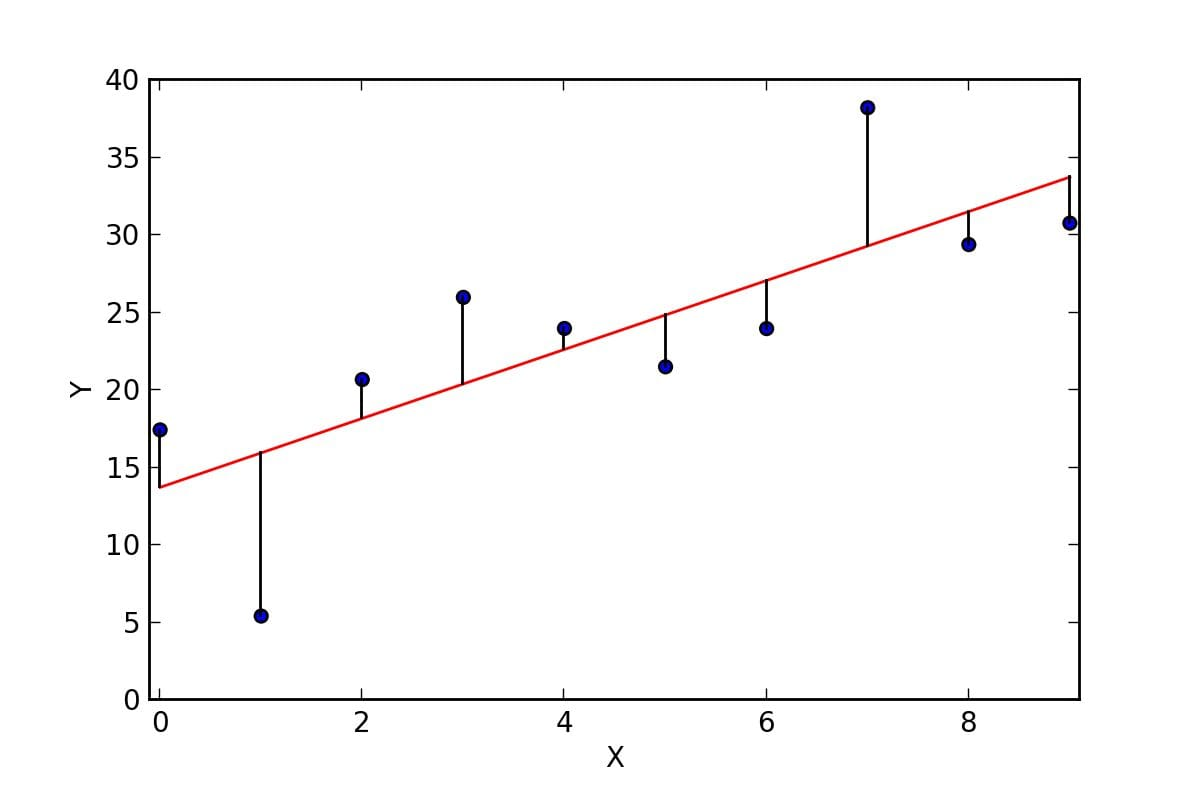# Linear Machine Learning Algorithms: An OverviewImage by Author

Linear machine learning algorithms assume a linear relationship between the features and the target variable. In this article, we’ll discuss several linear algorithms and their concepts. Here’s a glimpse into what you can expect to learn:

• Types of linear ML algorithms.
• Assumptions of linear algorithms.
• The difference between various linear machine learning algorithms.
• How to interpret the results of linear algorithms.
• When to use different linear algorithms.

Let’s dive right in.

# Types of Linear Machine Learning Algorithms

You can use linear algorithms for classification and regression problems. Let’s start by looking at different algorithms and what problems they solve.

## Linear regression

Linear regression is arguably one of the oldest and most popular algorithms. With roots in the statistics world, the algorithm is used for solving regression problems. This means that the final output of the model is a numeric value. The algorithm maps a linear relationship between the input features(X) and the output (y).

The Ordinary least squares Linear Regression is one the most widely used implementations of linear regression. It fits a linear model with coefficients `w = (w1, …, wp) to minimize the residual sum of squares between the observed targets in the dataset and the targets predicted by the linear approximation`. Source

In this approach, multiple lines are fitted, and the one that returns the least error is taken as the best fit. The error is the difference between the estimated value and the actual value.

Since linear regression fits a line, it can extrapolate to future data, unlike other algorithms such as random forests. Tree-based algorithms can not predict values outside the given data because their predictions are based on the mean of the predictions of different decision trees.Residuals for Linear Regression Fit

For example, when predicting the price of a commodity, a line is fitted between the independent and dependent variables. The general equation of a linear regression line looks like this:

where:

• c is the intercept, that is, the value of y when x is zero.
• m is the slope of the line.
• x is the independent variable.
• y is the dependent variable.

Other linear regression algorithms include:

• Lasso regression a linear model that introduces the L1 regularization whose objective is to minimize the absolute sum of the coefficients. In this algorithm, the weights of insignificant features are driven to zero. Hence insignificant features are dropped from the linear equation making the final equation simpler.
• Ridge regression whose coefficients minimize a penalized residual sum of squares. This regularization is referred to as L2 regularization. In this algorithm, the weights of insignificant features are reduced to small numbers close to zero but not zero. This is handy for trimming coefficients while keeping all features while adjusting the weights accordingly.

## Assumptions of linear regression

Linear regression is sensitive to outliers in the data. Other items to look out for include:

• The data has a normal distribution.
• That the relationship between the input variables and the output is linear.
• The variables are not highly correlated. In the event of this occurrence, remove highly correlated variables.
• Standardizing or normalizing the data also doesn’t hurt the algorithm!

## Logistic Regression

Logistic regression is a linear model for classification problems. It generates a probability between 0 and 1. This happens by fitting a logistic function, also known as the sigmoid function. The function is responsible for mapping the predicted values to numbers between 0 and 1.The Logistic Function | Image source

When used for multi-class classification, it is referred to as multinomial regression. This is done by adjusting the logistic regression algorithm to predict a multinormal distribution. It is achieved by changing the loss function used to train the model from log-loss to cross-entropy loss. The algorithm is also altered to output a probability for each class instead of a single probability.

One of the most popular implementations of Logistic Regression is done in Scikit-learn. This implementation handles imbalanced datasets by setting the `class_weight` parameter to `balanced.` In this case, the algorithm will adjust the weights to give more importance to the class with fewer samples, enabling the algorithm to learn more from that class.

Logistic regression assumptions

Logistic regression algorithm assumptions are similar to those of linear regression.  The unique addition here is that the algorithm expects the target variable to be categorical.

## Support Vector Machines

Support Vector Machines(SVM) is a supervised machine learning algorithm that can be used for regression and classification problems. It is mostly used for classification.

Linear SVM is used when data is linearly separable. In this case, the data is easily separated using a single line.

Classification is done by finding the hyperplane that best separates the two categories. SVM uses extreme data points in creating decision boundaries. These extreme data points are referred to as support vectors, hence the name Support Vector Machines.Support Vector Machine model. Support vectors are created to maximize the separation between two groups.

The distance between the vectors is known as a margin. The algorithm works by maximizing the gap between the two categories––the margin.  The optimal hyperplane is the hyperplane with the maximum margin.Maximum-margin hyperplane and margin for an SVM trained on two classes.

SVM can also compute boundaries for non-linear classification through a technique known as the kernel trick. It works by adding a third dimension and separating the data points in a 3D space.An illustration of kernel trick in SVM

# Difference Between SVM, Linear, and Logistic Regression

A linear regression algorithm is different from logistic regression in that the logistic regression outputs probabilities. Hence, the logistic regression algorithm can be used for classification while the linear regression algorithm can’t. While the logistic regression algorithm output values between 0 and 1, the linear regression algorithm will extrapolate and output values above and below zero.

SVM is more memory efficient because it uses a subset of the training data in the decision function. Unlike the logistic regression algorithm, SVM doesn’t provide probability estimates. SVM is also not prone to outliers like logistic regression because it is mainly concerned with the data points closest to the hyperplanes.

# Interpreting Linear Algorithm Results: Summary Statistics

A linear regression outputs a coefficient for each independent variable. Let’s take an example of an independent variable X1 used to predict the price of a commodity. If the coefficient of X1 is 6.2, it can be interpreted as follows; when all factors are held constant, a one-unit increase in F1 will lead to a  6.2 increase in the commodity's price.

A logistic regression algorithm outputs the probability of an item belonging to a certain class. Therefore, interpreting the results is done by setting a threshold of 0.5 to separate the two classes.

Given a certain dataset with two classes, the SVM algorithm will predict the class an item belongs to by computing the best hyperplane. It does not compute any probabilities but uses the distance of the data points from the optimal hyperplane to separate the categories.

# When to Use Logistic Regression vs. Support Vector Machines

Logistic regression and Support Vector Machines are two popular algorithms in classification. However, in certain situations, you might prefer one over the other. For instance, it’s better to use SVM when there are outliers because they don’t affect how the algorithm separates the two classes. SVM is also a better choice when the data is of high dimension.

Logistic regression is a better choice when classes are not well separated. Otherwise, use SVM. However, it’s common to start with a logistic regression model to create a baseline model.

# Final Thoughts

Linear algorithms are quite popular in machine learning. In this article, we have looked at a couple of linear models and their inner workings. We have also talked about when you would prefer one over the other. Generally, the choice of algorithm will depend on the problem and the dataset.

## Resources

Regularization for sparsity

Derrick Mwiti is experienced in data science, machine learning, and deep learning with a keen eye for building machine learning communities.